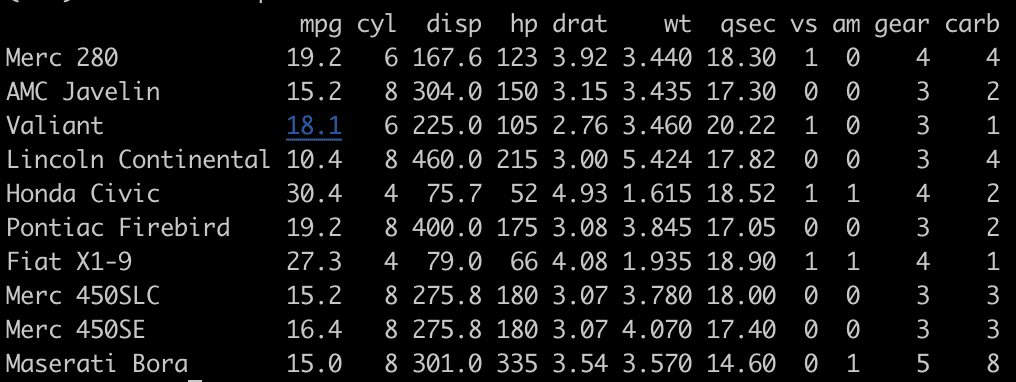# What is the sample() Function in R (5 Examples)

The sample() function in R allows you to take a random sample of elements from a dataset or vector. The data can be a vector or a dataframe. The sample() function can be used with or without replacement. By default, it is done without replacement.

## Syntax

``sample(data, size, replace = FALSE, prob = NULL)``

## Parameters

1. Data: It is either a vector of one or more elements from which to choose or a positive integer.
2. size: It is a non-negative integer giving the number of items to choose from.
3. replace: Should sampling be with replacement?
4. prob: It is a vector of probability weights for obtaining the elements of the sampled vector.

## Example 1: Generating a Sample from a Vector

Let’s define a numerical vector using :(colon operator) and sample the 5 values from that vector.

``````data <- 1:20
sample(data, 5, replace = FALSE, prob = NULL)``````

Output

`` 17 6 13 11 19``

## Example 2: Generating a Sample from a Dataset

We can generate a random sample of rows from a dataset. For the following example, we will generate a random sample of 10 rows from the built-in R dataset mtcars.

``````set.seed(100)

sample_rows <- sample(1:nrow(mtcars), 10)
sample <- mtcars[sample_rows, ]
sample``````

OutputLet’s work with a custom dataset.

``````# Set seed for reproducibility
set.seed(123)

# Create a dataset
data <- 1:100

# Generate a random sample of size 10 from the data
sample_data <- sample(data, size = 10)

# Print the sample
print(sample_data)
``````

Output

``  31 79 51 14 67 42 50 43 97 25``

## Example 3: Random Reordering of Data using sample() function

The most common usage of the sample function is the random subsampling of data. First, let’s subsample the vector.

``````rv <- 1:20

sample(rv, size = 10)``````

Output

`` 16  8  11  20  19  10  4  17  21  12``

## Example 4: Sampling with uneven probabilities using sample() function

To modify the probabilities of our random selection, pass the “prob” argument of the sample function.

``````rv <- 1:11

sample(rv, size = 10, replace = TRUE, prob = c(0.6, rep(0.1, 10)))``````

Output

``   1  11  1  1  5  3  10  1  1  2``

## Example 5: Random sampling of data frame rows

To extract the random subset of rows from a data frame in R, you can use the “sample()” function.

``````df <- data.frame(a1 = 1:10,
a2 = letters[1:10],
a3 = letters[1:10],
a4 = letters[1:10],
a5 = letters[1:10],
a6 = letters[1:10],
a7 = letters[1:10],
a8 = letters[1:10],
a9 = letters[1:10],
a10 = letters[1:10])

df_len <- length(df)

df_sample <- df[sample(seq_len(df_len), size = 3), ]

df_sample``````

Output

``````   a1  a2  a3  a4  a5  a6  a7  a8  a9  a10
8  8   h   h   h   h   h   h   h   h    h
1  1   a   a   a   a   a   a   a   a    a
10 10  j   j   j   j   j   j   j   j    j``````

That’s it.